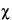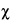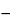Combination of Independent Assays When the method permits, additional animals can be added to an insufficiently precise assay until the combined results reduce the confidence interval within the limits specified in the monograph. Where two or more independent assays are required, each leading to a log-potency M, the M's are combined in determining the weighted mean potency of the Unknown. Except in the Heparin Sodium assay, where the log-potencies are weighted equally, the relative precisions of the two or more independent M's determine the weight assigned to each value in computing their mean and its confidence interval. Before combining two or more separate estimates of M, test their mutual consistency. If the M's are consistent, their respective confidence intervals will overlap. Where the intervals do not overlap or where the overlap is small, compute an approximateM2. Assign each of the h individual assays a weight w, defined as w = 4t2/L2(38) where the length of the confidence interval L is computed with the appropriate equation from the preceding section, and t2 is read from Table 9 for the degrees of freedom n in the error variance of the assay. Sum the individual weights to obtain Sw. Then an approximate2 with h – 1 degrees of freedom is determined as Approx.M2 = S(wM2){S(wM)}2/Sw(39) For two assays with log-potencies M1 and M2 and weights w1 and w2, Equation 35 reduces to Approx.M2 = w1w2(M1M2)2/(w1 + w2)(40) with one degree of freedom. If the approximateM2 is well under the critical value for2 in Table 9, use the weights w in computing the mean log-potency M and its confidence interval, L. IfM2 approaches or exceeds this critical value, use instead the semi-weights w¢ (Equation 47) when computing M. Compute the mean log-potency M of two or more mutually consistent assays as M = S(wM)/Sw(41) This is the most probable single value within a combined confidence interval of length Lc, defined as the square root of Lc2 = 4tL2/Sw {1 + (4/S2w)S(w(Sww)/n¢}(42) where each n¢ = n – 4(h – 2)/(h – 1) and tL2 is interpolated from Table 9 with the degrees of freedom nL = S2w/S(w2/n) For two assays (h = 2) with log-potencies M1 and M2 and weights w1 and w2, respectively, the above equation may be rewritten as Lc2 = 4tL2/Sw {1 + [1/n1 + 1/n2]4w1w2/S2w}(43) where Sw = w1 + w2. Where Lc, the confidence interval for a combined estimate, does not exceed the requirement in a monograph, upper and lower confidence limits are taken ½Lc above and below M, to obtain approximately a 95% confidence interval. Where the variation in the assayed potency between the h independent determinations, as tested byM2, approaches or exceeds P = 0.05, the several estimates are assigned semi-weights w¢. From the weight w, compute the variance of each M as V = 1/w = L2/4t2(44) Calculate the variance of the heterogeneity between assays as v = SM2(SM2/h)/(h1)Sv/h(45) or if h = 2, v = (M1M2)2/2(V1 + V2)/2(46) Where V varies so markedly that v calculated as above is a negative number, compute instead an approximate v by omitting the term following the minus sign in Equations 45 and 46. A semi-weight is defined as w¢ = 1/(V + v)(47) Substitute w¢ and Sw¢ for w and Sw in Equation 41 to obtain the semi-weighted mean M. This falls near the middle of a confidence interval of approximate length Lc¢, where Lc¢2 = 4t2/Sw¢(48) and t2 from Table 9 has SN degrees of freedom. WhereM2 in Equation 39, from h = 4 or more estimates of M, exceeds the critical level in Table 9 by more than 50%, and the weights w differ by less than 30%, the h estimates of M may be checked for a suspected outlier with Table 1. Where significant, the outlying M may be omitted in computing M with w¢. Where the potency of a drug is determined repeatedly in a given laboratory by the same bioassay method, successive determinations of both the slope b and the error variance s2 may scatter randomly within the sampling error about a common value for each parameter. Plotting estimates from successive assays on a quality control chart for each statistic and computing the midvalue and control limits defining the allowable random variation make it possible to check continuously the consistency of an assay technique. Where estimates of b and s2 from a single assay fall within the control limits, they may be replaced by their laboratory means. Reject any assay in which these statistics fall outside the control limits, or accept it only after close scrutiny with respect to its validity.